3.4 Primitives and Properties of Space

3.4 Primitives and Properties of Space

Within any coordinate space an infinite number of things can exist: shapes, points, lines, angles, and so on. These items and their mathematical relationships are discussed here and in subsequent sections.

3.4.1 Vertices

A point in a coordinate system is called a vertex (plural: vertices) and marks a point on the graph. Vertices are defined by coordinates.

3.4.2 Lines and Line Segments

In mathematics, a line is defined as being infinite-it has no beginning and no end. A line segment is a subset of a line. It has a beginning and an ending that are marked by two vertices, the start and end vertices. In other words, a line segment can be defined in coordinate space by two vertices.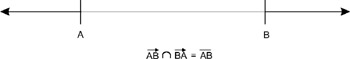Figure 3.4: Line segment

3.4.3 Ray

A ray is a line with only a starting point that extends infinitely in a straight line.

3.4.4 Angle

Two lines that cross are said to intersect, and the point where they meet is called the vertex of the angle. This is because, at this point, the lines form an angle to each other. An angle is the inclination one line has to the other; in other words, it is the measure of the amount of turn that makes one line, when rotated, fit onto the other. Angles are typically measured in degrees, with a complete turn (revolution) being 360, a half turn being 180, and a quarter turn being 90. As we shall see later in this chapter, there is another unit of angle measure that is used more commonly in game programming.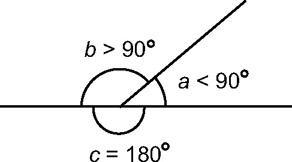Figure 3.5: Angles

3.4.4.1 Perpendicular

If one line intersects another such that it stands on top of the other and the adjacent angles are equal, then the lines are said to be perpendicular, or orthogonal. The two equal angles are each called right angles;in degrees, their measure is 90.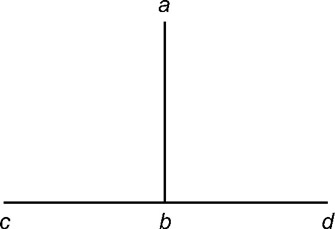Figure 3.6: Perpendicular lines

3.4.4.2 Angle Types

As mentioned, a right angle is a quarter turn and is 90 degrees. Other kinds of angles are defined in relation to right angles:

• An angle less than a right angle is said to be acute.

• An angle greater than a right angle is said to be obtuse.

• An angle greater than two right angles is said to be reflex.

• Two angles that together equal a right angle are called complementary angles.

• Two angles that added together equal two right angles are said to be supplementary angles.

3.4.5 Parallel

Parallel lines or line segments are two lines that never intersect. Even if they were extended infinitely, there would be no point at which they cross.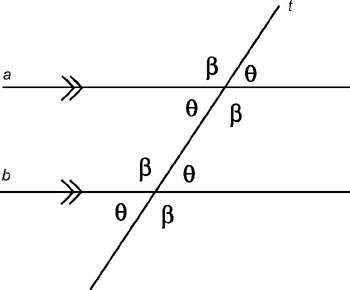Figure 3.7: Parallel lines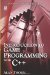Introduction to Game Programming with C++ (Wordware Game Developers Library)
ISBN: 1598220322
EAN: 2147483647
Year: 2007
Pages: 225
Authors: Alan Thorn

Similar book on Amazon